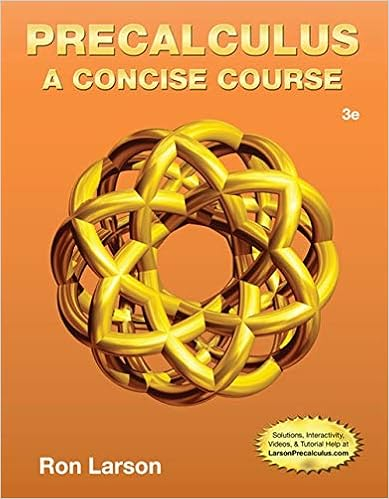# 49 r 2 81t 2 2factor completely 200 18 n2 3 factor

• Notes
• 74

This preview shows page 27 - 31 out of 74 pages.

##### We have textbook solutions for you!
The document you are viewing contains questions related to this textbook.The document you are viewing contains questions related to this textbook.
Chapter 2 / Exercise 55
Precalculus: A Concise Course
LarsonExpert Verified
Factor completely.49r281t22.Factor completely.200 18n23.Factor completely.3t2+ 754.Factor completely.16h2015.Factor completely.(c+ d)225Factor By Grouping (3 Terms + 1 Term)Perfect Square Trinomials:))((222BABABABA))((222BABABABA6.Factor completely.w212w+ 36 64z227 | P a g e
##### We have textbook solutions for you!
The document you are viewing contains questions related to this textbook.The document you are viewing contains questions related to this textbook.
Chapter 2 / Exercise 55
Precalculus: A Concise Course
LarsonExpert Verified
7.Factor completely.121 + 44p+ 4p249q28.Factor completely.25c230cd+ 9d 2144Factoring Sums or Differences of Cubes 9.Factor completely.n3+ 6410.Factor completely.8x3125 11.Factor completely.343 w1512.Factor completely.54p6+ 16q3Preparation for Lesson 10: Quadratic and Cubic Equations by FactoringI earned ______ pts out of 5ptsDue Date: _________________Video Assignment #10(8 videos)a) Fold paper in half (down the center vertically)b) On the left side of the paper: Write the question & title. (Ex: Video #1 - Problem #1 McKeague) c) Watch the video & copy the full video play. Notes must be clear, neat, and legible.d) On the right side of the paper: Write the highlights of the video such as important facts, definitions, formulas, properties and rules. Rate the video. Video #1:MathTV.com Algebra Factoring Quadratic EquationsFactoring Problem #1 Mr. McKeague (2:19 mins)Video #2:MathTV.com Algebra Factoring Quadratic EquationsFactoring Problem #4 Mr. McKeague (3:09 mins)Video #3:MathTV.com Algebra Factoring Quadratic EquationsFactoring Problem #6 Mr. McKeague (2:26 mins)Video #4:MathTV.com Algebra Factoring Quadratic EquationsFactoring Problem #8 Mr. McKeague (2:10 mins)Video #5:MathTV.com Algebra Factoring Quadratic EquationsFactoring Problem #10 Mr. McKeague (2:06 mins)Video #6:MathTV.com Algebra Factoring Quadratic EquationsFactoring Problem #11 Mr. McKeague (3:06 mins)Video #7:MathTV.com Algebra Factoring Quadratic EquationsFactoring Problem #12 Graham (2:27 minutes)YouTube Video #8: Solving Quadratic Equations by Factoring - Basic Examples(7:18 minutes)28 | P a g e
Video Follow-Up Mini Exercise Set #10: Directions: On binder paper, write down each question, answer fully, show every step, and circle your final answer.1.Solve.3p3+ 2p275p50 = 02.Solve.(r 2) (r 9) = 44 3.Solve.a21.7a+ 0.3 = 04.Solve.451213212nnQuote of the DayAll our dreams can cometrue, if we have the courageto pursue them.- Walt DisneyMAT 1033-Intermediate AlgebraCreated by Alice WongLesson 10: Solving Quadratic and Cubic Equations by FactoringQuadratic Equations (in one variable)= Any equation that can be written in the form 0,,02AandnumbersrealareCBAwhereCBxAx(Standard Form)Cubic Equations (in one variable)= Any equation that can be written in the form0,,,023AandnumbersrealareDandCBAwhereDCxBxAx(Standard Form)Zero-Factor PropertyIf A B = 0, then A = 0 or B = 01.Solve.5k2+ 30 = 35k2.Solve.7m2= 63m29 | P a g e
3.Solve.4h320h2= 9h454.Solve.
•••